# HE plot MANOVA Examples

#### 2023-09-04

Vignette built using heplots, version 1.6.0 and candisc, version 0.8-6.

# Multivariate Analysis of Variance Designs

This vignette provides some worked examples of the analysis of multivariate linear models (MLMs) for MANOVA designs where all predictors are factors, and the goal is to determine how the group means differ on several response variables in relation to the factors and possible interactions.

Graphical methods for visualizing results using the heplots and the candisc packages are illustrated. The emphasis here is on using these methods in R, and understanding how they help reveal aspects of these models that might not be apparent from other graphical displays.

No attempt is made here to describe the theory of MLMs or the statistical details behind HE plots and their reduced-rank canonical cousins. For that, see Fox, Friendly, & Monette (2009); Friendly (2007); Friendly (2006).

# 1 Plastic film data

An experiment was conducted to determine the optimum conditions for extruding plastic film. Three responses, tear resistance, film gloss and film opacity were measured in relation to two factors, rate of extrusion and amount of an additive, both of these being set to two values, High and Low. The data set comes from Johnson & Wichern (1992).

data(Plastic, package="heplots")
str(Plastic)
#> 'data.frame':    20 obs. of  5 variables:
#>  $tear : num 6.5 6.2 5.8 6.5 6.5 6.9 7.2 6.9 6.1 6.3 ... #>$ gloss   : num  9.5 9.9 9.6 9.6 9.2 9.1 10 9.9 9.5 9.4 ...
#>  $opacity : num 4.4 6.4 3 4.1 0.8 5.7 2 3.9 1.9 5.7 ... #>$ rate    : Factor w/ 2 levels "Low","High": 1 1 1 1 1 1 1 1 1 1 ...
#>  $additive: Factor w/ 2 levels "Low","High": 1 1 1 1 1 2 2 2 2 2 ... The design is thus a $$2\times 2$$ MANOVA, with $$n=5$$ per cell and 3 numeric response variables. Because the effects of the factors on the responses are likely correlated, it is useful to consider a multivariate analysis, rather than 3 separate univariate ones. This example illustrates: • 2D and 3D HE plots, • the difference between “effect” scaling and “evidence” (significance) scaling, and • visualizing composite linear hypotheses. We begin with an overall MANOVA for the two-way MANOVA model. In all these analyses, we use car::Anova() for significance tests rather than stats::anova(), which only provides so-called “Type I” (sequential) tests for terms in linear models. In this example, because each effect has 1 df, all of the multivariate statistics (Roy’s maximum root test, Pillai and Hotelling trace criteria, Wilks’ Lambda) are equivalent, in that they give the same $$F$$ statistics and $$p$$-values. We specify test.statistic="Roy" to emphasize that Roy’s test has a natural visual interpretation in HE plots. plastic.mod <- lm(cbind(tear, gloss, opacity) ~ rate*additive, data=Plastic) Anova(plastic.mod, test.statistic="Roy") #> #> Type II MANOVA Tests: Roy test statistic #> Df test stat approx F num Df den Df Pr(>F) #> rate 1 1.619 7.55 3 14 0.003 ** #> additive 1 0.912 4.26 3 14 0.025 * #> rate:additive 1 0.287 1.34 3 14 0.302 #> --- #> Signif. codes: 0 '***' 0.001 '**' 0.01 '*' 0.05 '.' 0.1 ' ' 1 For the three responses jointly, the main effects of rate and additive are significant, while their interaction is not. In some approaches to testing effects in multivariate linear models (MLMs), significant multivariate tests are often followed by univariate tests on each of the responses separately to determine which responses contribute to each significant effect. In R, univariate analyses are conveniently performed using the update() method for the mlm object plastic.mod, which re-fits the model with only a single outcome variable. Anova(update(plastic.mod, tear ~ .)) #> Anova Table (Type II tests) #> #> Response: tear #> Sum Sq Df F value Pr(>F) #> rate 1.74 1 15.8 0.0011 ** #> additive 0.76 1 6.9 0.0183 * #> rate:additive 0.00 1 0.0 0.9471 #> Residuals 1.76 16 #> --- #> Signif. codes: 0 '***' 0.001 '**' 0.01 '*' 0.05 '.' 0.1 ' ' 1 Anova(update(plastic.mod, gloss ~ .)) #> Anova Table (Type II tests) #> #> Response: gloss #> Sum Sq Df F value Pr(>F) #> rate 1.301 1 7.92 0.012 * #> additive 0.612 1 3.73 0.071 . #> rate:additive 0.544 1 3.32 0.087 . #> Residuals 2.628 16 #> --- #> Signif. codes: 0 '***' 0.001 '**' 0.01 '*' 0.05 '.' 0.1 ' ' 1 Anova(update(plastic.mod, opacity ~ .)) #> Anova Table (Type II tests) #> #> Response: opacity #> Sum Sq Df F value Pr(>F) #> rate 0.4 1 0.10 0.75 #> additive 4.9 1 1.21 0.29 #> rate:additive 4.0 1 0.98 0.34 #> Residuals 64.9 16 The results above show significant main effects for tear, a significant main effect of rate for gloss, and no significant effects for opacity, but they don’t shed light on the nature of these effects. Traditional univariate plots of the means for each variable separately are useful, but they don’t allow visualization of the relations among the response variables. We can visualize these effects for pairs of variables in an HE plot, showing the “size” and orientation of hypothesis variation ($$\mathbf{H}$$) in relation to error variation ($$\mathbf{E}$$) as ellipsoids. When, as here, the model terms have 1 degree of freedom, the $$\mathbf{H}$$ ellipsoids degenerate to a line. In HE plots, the $$\mathbf{H}$$ ellipses can be scaled relative to the $$\mathbf{E}$$ to show significance of effects (size="evidence"), or effect size (size="effect"). In the former case, a model term is significant (using Roy’s maximum root test) iff the $$\mathbf{H}$$ projects anywhere outside the $$\mathbf{E}$$ ellipse. This plot overlays those for both scaling, using thicker lines for the effect scaling. ## Compare evidence and effect scaling colors = c("red", "darkblue", "darkgreen", "brown") heplot(plastic.mod, size="evidence", col=colors, cex=1.25, fill=TRUE, fill.alpha=0.1) heplot(plastic.mod, size="effect", add=TRUE, lwd=5, term.labels=FALSE, col=colors)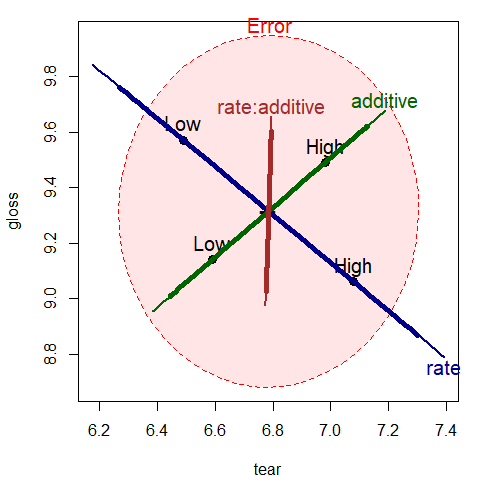Figure 1.1: HE plot for effects on tear and gloss according to the factors rate, additive and their interaction, rate:additive. The thicker lines show effect size scaling; the thinner lines show significance scaling. The interpretation can be easily read from the plot, at least for the two response variables (tear and gloss) that are shown in this bivariate view. The effect of rate of extrusion is highly significant: high rate shows greater tear compared to low rate. The effect of amount of additive is not significant in this view, but high level of additive has greater tear and gloss. With effect scaling, both the $$\mathbf{H}$$ and $$\mathbf{E}$$ sums of squares and products matrices are both divided by the error df, giving multivariate analogs of univariate measures of effect size, e.g., $$(\bar{y}_1-\bar{y}_2) / s$$. With significance scaling, the $$\mathbf{H}$$ ellipse is further divided by $$\lambda_\alpha$$, the critical value of Roy’s largest root statistic. This scaling has the property that an $$\mathbf{H}$$ ellipse will protrude somewhere outside the $$\mathbf{E}$$ ellipse iff the multivariate test is significant at level $$\alpha$$. Figure 1.2 shows both scalings, using a thinner line for significance scaling. Note that the (degenerate) ellipse for additive is significant, but does not protrude outside the $$\mathbf{E}$$ ellipse in this view. All that is guaranteed is that it will protrude somewhere in the 3D space of the responses. By design, means for the levels of interaction terms are not shown in the HE plot, because doing so in general can lead to messy displays. We can add them here for the term rate:additive as follows: # Compare evidence and effect scaling colors = c("red", "darkblue", "darkgreen", "brown") heplot(plastic.mod, size="evidence", col=colors, cex=1.25, fill=TRUE, fill.alpha=0.05) heplot(plastic.mod, size="effect", add=TRUE, lwd=5, term.labels=FALSE, col=colors) ## add interaction means intMeans <- termMeans(plastic.mod, 'rate:additive', abbrev.levels=2) points(intMeans[,1], intMeans[,2], pch=18, cex=1.2, col="brown") text(intMeans[,1], intMeans[,2], rownames(intMeans), adj=c(0.5, 1), col="brown") lines(intMeans[c(1,3),1], intMeans[c(1,3),2], col="brown") lines(intMeans[c(2,4),1], intMeans[c(2,4),2], col="brown")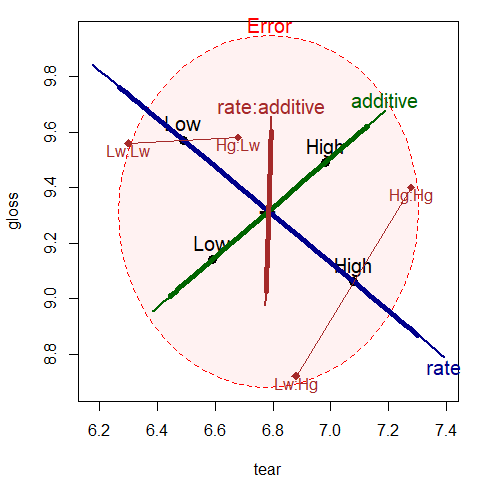Figure 1.2: HE plot for effects on tear and gloss according to the factors rate, additive and their interaction, rate:additive. Annotations have added means for the combinations of rate and additive. The factor means in this plot (Figure 1.2 have a simple interpretation: The high rate level yields greater tear resistance but lower gloss than the low level. The high additive amount produces greater tear resistance and greater gloss. The rate:additive interaction is not significant overall, though it approaches significance for gloss. The cell means for the combinations of rate and additive shown in this figure suggest an explanation, for tutorial purposes: with the low level of rate, there is little difference in gloss for the levels of additive. At the high level of rate, there is a larger difference in gloss. The $$\mathbf{H}$$ ellipse for the interaction of rate:additive therefore “points” in the direction of gloss indicating that this variable contributes to the interaction in the multivariate tests. In some MANOVA models, it is of interest to test sub-hypotheses of a given main effect or interaction, or conversely to test composite hypotheses that pool together certain effects to test them jointly. All of these tests (and, indeed, the tests of terms in a given model) are carried out as tests of general linear hypotheses in the MLM. In this example, it might be useful to test two composite hypotheses: one corresponding to both main effects jointly, and another corresponding to no difference among the means of the four groups (equivalent to a joint test for the overall model). These tests are specified in terms of subsets or linear combinations of the model parameters. plastic.mod #> #> Call: #> lm(formula = cbind(tear, gloss, opacity) ~ rate * additive, data = Plastic) #> #> Coefficients: #> tear gloss opacity #> (Intercept) 6.30 9.56 3.74 #> rateHigh 0.58 -0.84 -0.60 #> additiveHigh 0.38 0.02 0.10 #> rateHigh:additiveHigh 0.02 0.66 1.78 Thus, for example, the joint test of both main effects tests the parameters rateHigh and additiveHigh. print(linearHypothesis(plastic.mod, c("rateHigh", "additiveHigh"), title="Main effects"), SSP=FALSE) #> #> Multivariate Tests: Main effects #> Df test stat approx F num Df den Df Pr(>F) #> Pillai 2 0.71161 2.7616 6 30 0.029394 * #> Wilks 2 0.37410 2.9632 6 28 0.022839 * #> Hotelling-Lawley 2 1.44400 3.1287 6 26 0.019176 * #> Roy 2 1.26253 6.3127 3 15 0.005542 ** #> --- #> Signif. codes: 0 '***' 0.001 '**' 0.01 '*' 0.05 '.' 0.1 ' ' 1 print(linearHypothesis(plastic.mod, c("rateHigh", "additiveHigh", "rateHigh:additiveHigh"), title="Groups"), SSP=FALSE) #> #> Multivariate Tests: Groups #> Df test stat approx F num Df den Df Pr(>F) #> Pillai 3 1.14560 3.2948 9 48.000 0.003350 ** #> Wilks 3 0.17802 3.9252 9 34.223 0.001663 ** #> Hotelling-Lawley 3 2.81752 3.9654 9 38.000 0.001245 ** #> Roy 3 1.86960 9.9712 3 16.000 0.000603 *** #> --- #> Signif. codes: 0 '***' 0.001 '**' 0.01 '*' 0.05 '.' 0.1 ' ' 1 Correspondingly, we can display these tests in the HE plot by specifying these tests in the hypothesis argument to heplot(), as shown in Figure 1.3. heplot(plastic.mod, hypotheses=list("Group" = c("rateHigh", "additiveHigh", "rateHigh:additiveHigh ")), col=c(colors, "purple"), fill = TRUE, fill.alpha = 0.1, lwd=c(2, 3, 3, 3, 2), cex=1.25) heplot(plastic.mod, hypotheses=list("Main effects" = c("rateHigh", "additiveHigh")), add=TRUE, col=c(colors, "darkgreen"), cex=1.25)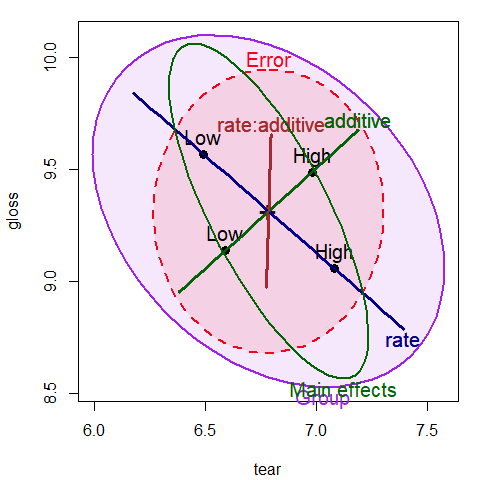Figure 1.3: HE plot for tear and gloss, supplemented with ellipses representing the joint tests of main effects and all group differences Finally, a 3D HE plot can be produced with heplot3d(), giving Figure 1.4. This plot was rotated interactively to a view that shows both main effects protruding outside the error ellipsoid. colors = c("pink", "darkblue", "darkgreen", "brown") heplot3d(plastic.mod, col=colors)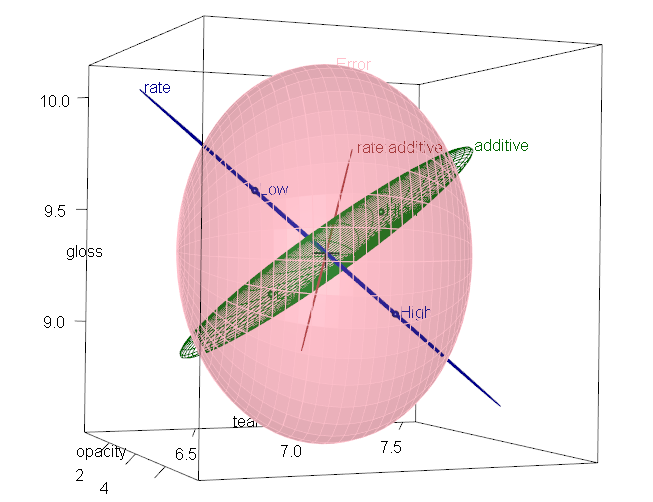Figure 1.4: 3D HE plot for the plastic MLM # 2 Effects of physical attractiveness on mock jury decisions In a social psychology study of influences on jury decisions by Plaster (1989), male participants (prison inmates) were shown a picture of one of three young women. Pilot work had indicated that one woman was beautiful, another of average physical attractiveness, and the third unattractive. Participants rated the woman they saw on each of twelve attributes on scales of 1–9. These measures were used to check on the manipulation of “attractiveness” by the photo. Then the participants were told that the person in the photo had committed a Crime, and asked to rate the seriousness of the crime and recommend a prison sentence, in Years. The data are contained in the data frame MockJury.1 data(MockJury, package = "heplots") str(MockJury) #> 'data.frame': 114 obs. of 17 variables: #>$ Attr         : Factor w/ 3 levels "Beautiful","Average",..: 1 1 1 1 1 1 1 1 1 1 ...
#>  $Crime : Factor w/ 2 levels "Burglary","Swindle": 1 1 1 1 1 1 1 1 1 1 ... #>$ Years        : int  10 3 5 1 7 7 3 7 2 3 ...
#>  $Serious : int 8 8 5 3 9 9 4 4 5 2 ... #>$ exciting     : int  6 9 3 3 1 1 5 4 4 6 ...
#>  $calm : int 9 5 4 6 1 5 6 9 8 8 ... #>$ independent  : int  9 9 6 9 5 7 7 2 8 7 ...
#>  $sincere : int 8 3 3 8 1 5 6 9 7 5 ... #>$ warm         : int  5 5 6 8 8 8 7 6 1 7 ...
#>  $phyattr : int 9 9 7 9 8 8 8 5 9 8 ... #>$ sociable     : int  9 9 4 9 9 9 7 2 1 9 ...
#>  $kind : int 9 4 2 9 4 5 5 9 5 7 ... #>$ intelligent  : int  6 9 4 9 7 8 7 9 9 9 ...
#>  $strong : int 9 5 5 9 9 9 5 2 7 5 ... #>$ sophisticated: int  9 5 4 9 9 9 6 2 7 6 ...
#>  $happy : int 5 5 5 9 8 9 5 2 6 8 ... #>$ ownPA        : int  9 7 5 9 7 9 6 5 3 6 ...

Sample sizes were roughly balanced for the independent variables in the three conditions of the attractiveness of the photo, and the combinations of this with Crime:

table(MockJury$Attr) #> #> Beautiful Average Unattractive #> 39 38 37 table(MockJury$Attr, MockJuryCrime) #> #> Burglary Swindle #> Beautiful 21 18 #> Average 18 20 #> Unattractive 20 17 The main questions of interest were: • Does attractiveness of the “defendant” influence the sentence or perceived seriousness of the crime? • Does attractiveness interact with the nature of the crime? ## 2.1 Manipulation check But first, as a check on the manipulation of attractiveness, we try to assess the ratings of the photos in relation to the presumed categories of the independent variable Attr. The questions here are: • do the ratings of the photos on physical attractiveness (phyattr) confirm the original classification? • how do other ratings differentiate the photos? To keep things simple, we consider only a few of the other ratings in a one-way MANOVA. (jury.mod1 <- lm( cbind(phyattr, happy, independent, sophisticated) ~ Attr, data=MockJury)) #> #> Call: #> lm(formula = cbind(phyattr, happy, independent, sophisticated) ~ #> Attr, data = MockJury) #> #> Coefficients: #> phyattr happy independent sophisticated #> (Intercept) 8.282 5.359 6.410 6.077 #> AttrAverage -4.808 0.430 0.537 -1.340 #> AttrUnattractive -5.390 -1.359 -1.410 -1.753 Anova(jury.mod1, test="Roy") #> #> Type II MANOVA Tests: Roy test statistic #> Df test stat approx F num Df den Df Pr(>F) #> Attr 2 1.77 48.2 4 109 <2e-16 *** #> --- #> Signif. codes: 0 '***' 0.001 '**' 0.01 '*' 0.05 '.' 0.1 ' ' 1 Note that Beautiful is the baseline category of Attr, so the intercept term gives the means for this level. We see that the means are significantly different on all four variables collectively, by a joint multivariate test. A traditional analysis might follow up with univariate ANOVAs for each measure separately. As an aid to interpretation of the MANOVA results We can examine the test of Attr in this model with an HE plot for pairs of variables, e.g., for phyattr and happy (Figure 2.1). The means in this plot show that Beautiful is rated higher on physical attractiveness than the other two photos, while Unattractive is rated less happy than the other two. Comparing the sizes of the ellipses, differences among group means on physical attractiveness contributes more to significance than do ratings on happy. heplot(jury.mod1, main="HE plot for manipulation check", fill = TRUE, fill.alpha = 0.1)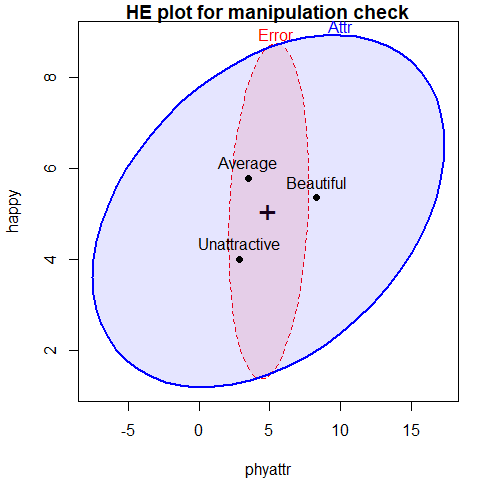Figure 2.1: HE plot for ratings of phyattr and happy according to the classification of photos on Attr The function pairs.mlm() produces all pairwise HE plots. This plot (Figure 2.2) shows that the means for happy and independent are highly correlated, as are the means for phyattr and sophisticated. In most of these pairwise plots, the means form a triangle rather than a line, suggesting that these attributes are indeed measuring different aspects of the photos. pairs(jury.mod1)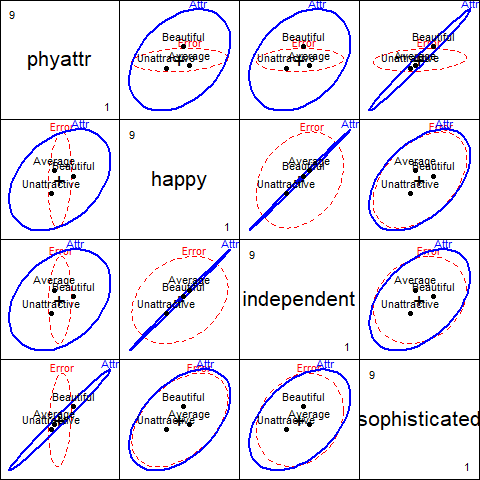Figure 2.2: HE plots for all pairs of ratings according to the classification of photos on Attr With 3 groups and 4 variables, the $$\mathbf{H}$$ ellipsoid has only $$s=\min(df_h, p)=2$$ dimensions. candisc() carries out a canonical discriminant analysis for the MLM and returns an object that can be used to show an HE plot in the space of the canonical dimensions. This is plotted in Figure 2.3. jury.can <- candisc(jury.mod1) jury.can #> #> Canonical Discriminant Analysis for Attr: #> #> CanRsq Eigenvalue Difference Percent Cumulative #> 1 0.639 1.767 1.6 91.33 91.3 #> 2 0.144 0.168 1.6 8.67 100.0 #> #> Test of H0: The canonical correlations in the #> current row and all that follow are zero #> #> LR test stat approx F numDF denDF Pr(> F) #> 1 0.309 21.53 8 216 < 2e-16 *** #> 2 0.856 6.09 3 109 0.00072 *** #> --- #> Signif. codes: 0 '***' 0.001 '**' 0.01 '*' 0.05 '.' 0.1 ' ' 1 heplot.candisc() is the HE plot method for candisc objects heplot(jury.can, rev.axes = TRUE, fill = c(TRUE,FALSE), prefix="Canonical dimension", main="Canonical HE plot")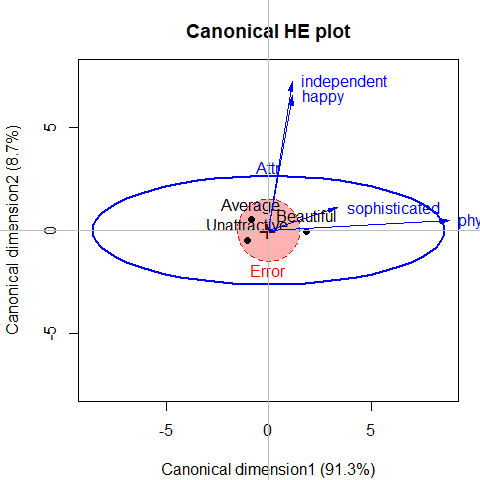Figure 2.3: Canonical discriminant HE plot for the MockJury data. Variable vectors show the correlations of the predictors with the canonical dimensions. #> Vector scale factor set to 8.8177 In this plot, • the variable vectors are determined by the canonical structure coefficients and represent the correlations of the predictor variables with the canonical variables. Thus, an angle near zero with an axis represents a correlation close to 1.0; an angle near 90$$^o$$ represent a correlation close to 0.0. (The axes must be scaled to have equal unit lengths for angles to be interpretable.) • The lengths of arrows are scaled to roughly fill the plot, but relative length represents the overall strength of the relation of the variable with the canonical dimensions. • Points represent the means of the canonical scores on the two dimensions for the three groups of photos. From this we can see that 91% of the variation among group means is accounted for by the first dimension, and this is nearly completely aligned with phyattr. The second dimension, accounting for the remaining 9% is determined nearly entirely by ratings on happy and independent. This display gives a relatively simple account of the results of the MANOVA and the relations of each of the ratings to discrimination among the photos. ## 2.2 Main analysis Proceeding to the main questions of interest, we carry out a two-way MANOVA of the responses Years and Serious in relation to the independent variables Attr and Crime. # influence of Attr of photo and nature of crime on Serious and Years jury.mod2 <- lm( cbind(Serious, Years) ~ Attr * Crime, data=MockJury) Anova(jury.mod2, test="Roy") #> #> Type II MANOVA Tests: Roy test statistic #> Df test stat approx F num Df den Df Pr(>F) #> Attr 2 0.0756 4.08 2 108 0.020 * #> Crime 1 0.0047 0.25 2 107 0.778 #> Attr:Crime 2 0.0501 2.71 2 108 0.071 . #> --- #> Signif. codes: 0 '***' 0.001 '**' 0.01 '*' 0.05 '.' 0.1 ' ' 1 We see that there is a nearly significant interaction between Attr and Crime and a strong effect of Attr. heplot(jury.mod2)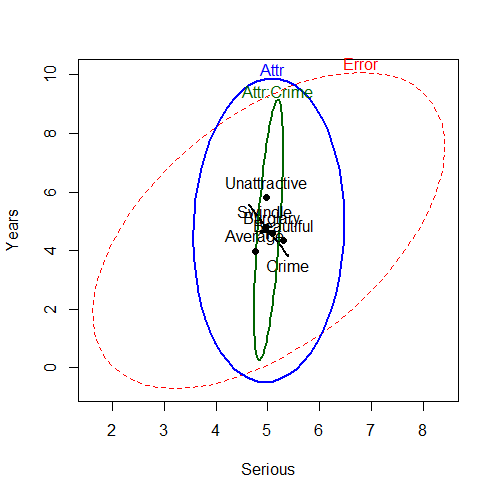Figure 2.4: HE plot for the two-way MANOVA for Years and Serious The HE plot shows that the nearly significant interaction of Attr:Crime is mainly in terms of differences among the groups on the response of Years of sentence, with very little contribution of Serious. We explore this interaction in a bit more detail below. The main effect of Attr is also dominated by differences among groups on Years. If we assume that Years of sentence is the main outcome of interest, it also makes sense to carry out a step-down test of this variable by itself, controlling for the rating of seriousness (Serious) of the crime. The model jury.mod3 below is equivalent to an ANCOVA for Years. # stepdown test (ANCOVA), controlling for Serious jury.mod3 <- lm( Years ~ Serious + Attr * Crime, data=MockJury) t(coef(jury.mod3)) #> (Intercept) Serious AttrAverage AttrUnattractive CrimeSwindle #> [1,] 0.011612 0.83711 0.39586 0.60285 -0.26302 #> AttrAverage:CrimeSwindle AttrUnattractive:CrimeSwindle #> [1,] -0.53701 2.5123 Anova(jury.mod3) #> Anova Table (Type II tests) #> #> Response: Years #> Sum Sq Df F value Pr(>F) #> Serious 379 1 41.14 3.9e-09 *** #> Attr 74 2 4.02 0.021 * #> Crime 4 1 0.43 0.516 #> Attr:Crime 49 2 2.67 0.074 . #> Residuals 987 107 #> --- #> Signif. codes: 0 '***' 0.001 '**' 0.01 '*' 0.05 '.' 0.1 ' ' 1 Thus, even when adjusting for Serious rating, there is still a significant main effect of Attr of the photo, but also a hint of an interaction of Attr with Crime. The coefficient for Serious indicates that participants awarded 0.84 additional years of sentence for each 1 unit step on the scale of seriousness of crime. A particularly useful method for visualizing the fitted effects in such univariate response models is provided by the effects. By default allEffects() calculates the predicted values for all high-order terms in a given model, and the plot method produces plots of these values for each term. The statements below produce Figure 2.5. library(effects) jury.eff <- allEffects(jury.mod3) plot(jury.eff, ask=FALSE)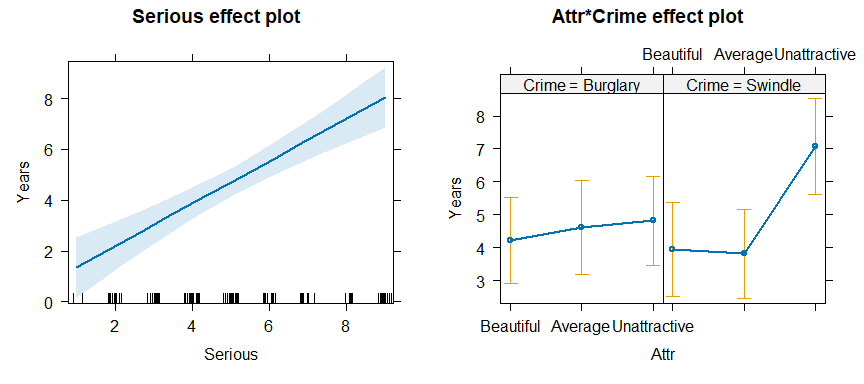Figure 2.5: Effect plots for Serious and the Attr * Crime interaction in the ANCOVA model jury.mod3. The effect plot for Serious shows the expected linear relation between that variable and Years. Of greater interest here is the nature of the possible interaction of Attr and Crime on Years of sentence, controlling for Serious. The effect plot shows that for the crime of Swindle, there is a much greater Years of sentence awarded to Unattractive defendants. # 3 Egyptian skulls from five epochs This example examines physical measurements of size and shape made on 150 Egyptian skulls from five epochs ranging from 4000 BC to 150 AD. The measures are: maximal breadth (mb), basibregmatic height (bh), basialiveolar length (bl), and nasal height (nh) of each skull. See Figure 3.1 for a diagram. The question of interest is whether and how these measurements change over time. Systematic changes over time is of interest because this would indicate interbreeding with immigrant populations.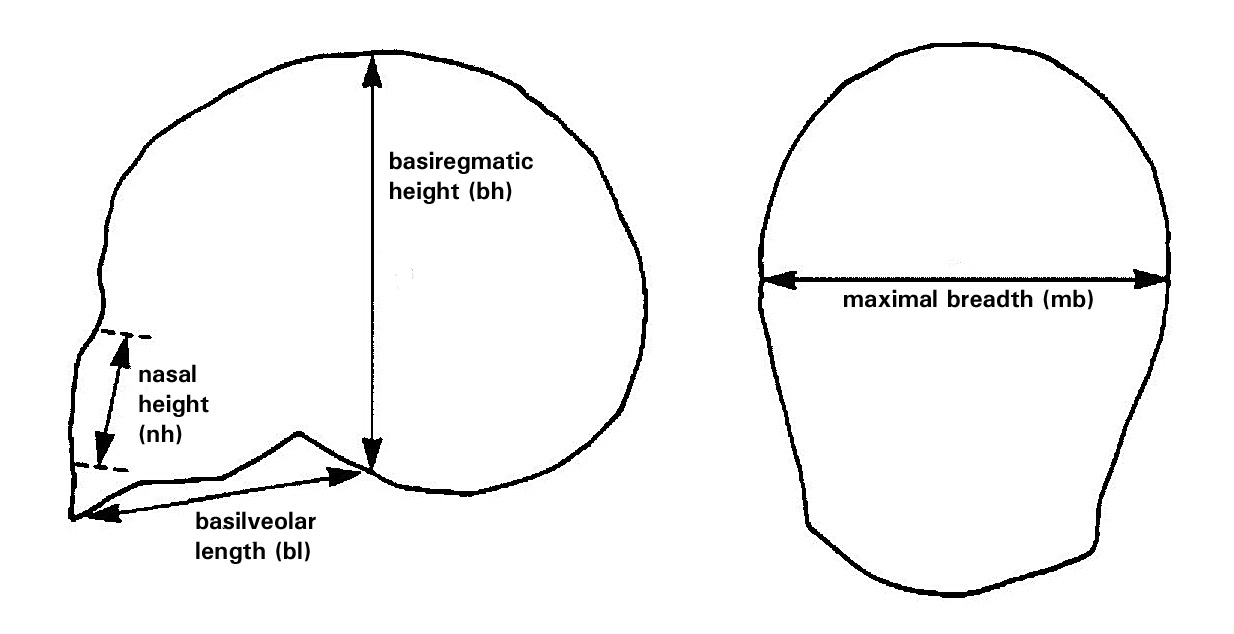Figure 3.1: Diagram of the skull measurements. Maximal breadth and basibregmatic height are the basic measures of “size” of a skull. Basialveolar length and nasal height are important anthropometric measures of “shape”. data(Skulls) str(Skulls) #> 'data.frame': 150 obs. of 5 variables: #> epoch: Ord.factor w/ 5 levels "c4000BC"<"c3300BC"<..: 1 1 1 1 1 1 1 1 1 1 ...
#>  $mb : num 131 125 131 119 136 138 139 125 131 134 ... #>$ bh   : num  138 131 132 132 143 137 130 136 134 134 ...
#>  $bl : num 89 92 99 96 100 89 108 93 102 99 ... #>$ nh   : num  49 48 50 44 54 56 48 48 51 51 ...
table(Skulls$epoch) #> #> c4000BC c3300BC c1850BC c200BC cAD150 #> 30 30 30 30 30 Note that epoch is an ordered factor, so the default contrasts will be orthogonal polynomials. This assumes that epoch values are equally spaced, which they are not. However, examining the linear and quadratic trends is useful to a first approximation. For ease of labeling various outputs, it is useful to trim the epoch values and assign more meaningful variable labels. # make shorter labels for epochs Skulls$epoch <- factor(Skulls$epoch, labels=sub("c","",levels(Skulls$epoch)))
# assign better variable labels
vlab <- c("maxBreadth", "basibHeight", "basialLength", "nasalHeight")

We start with some simple displays of the means by epoch. From the numbers, the means don’t seem to vary much.
A pairs plot, Figure 3.2, joining points by epoch is somewhat more revealing for the bivariate relations among means.

means <- aggregate(cbind(mb, bh, bl, nh) ~ epoch, data=Skulls, FUN=mean)[,-1]
rownames(means) <- levels(Skulls$epoch) means #> mb bh bl nh #> 4000BC 131.37 133.60 99.167 50.533 #> 3300BC 132.37 132.70 99.067 50.233 #> 1850BC 134.47 133.80 96.033 50.567 #> 200BC 135.50 132.30 94.533 51.967 #> AD150 136.17 130.33 93.500 51.367 pairs(means, vlab, panel = function(x, y) { text(x, y, levels(Skulls$epoch))
lines(x,y)
})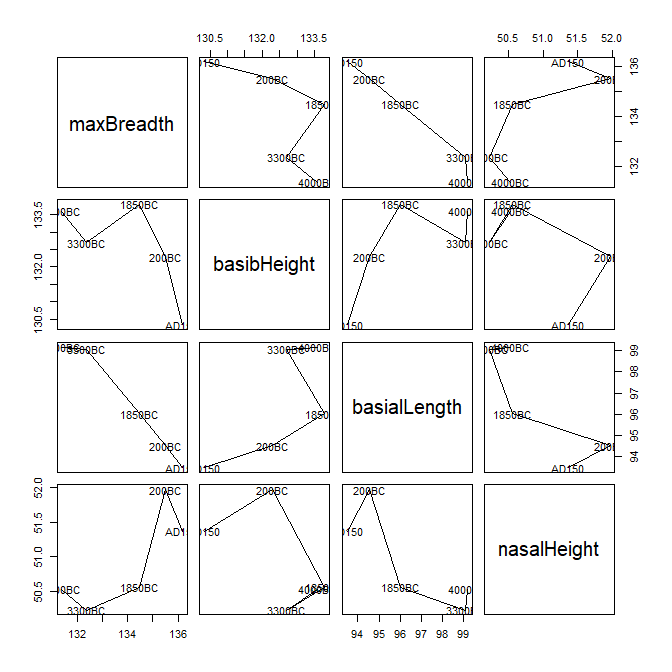Figure 3.2: Pairs plot of means of Skulls data, by epoch.

Perhaps better for visualizing the trends over time is a set of boxplots, joining means over epoch. Using bwplot() from the lattice package requires reshaping the data from wide to long format. The following code produces Figure 3.3.

library(lattice)
library(reshape2)
sklong <- melt(Skulls, id="epoch")

bwplot(value ~ epoch | variable, data=sklong, scales="free",
ylab="Variable value", xlab="Epoch",
strip=strip.custom(factor.levels=paste(vlab,
" (", levels(sklong\$variable), ")",
sep="")),
panel = function(x,y, ...) {
panel.bwplot(x, y, ...)
panel.linejoin(x,y, col="red", ...)
})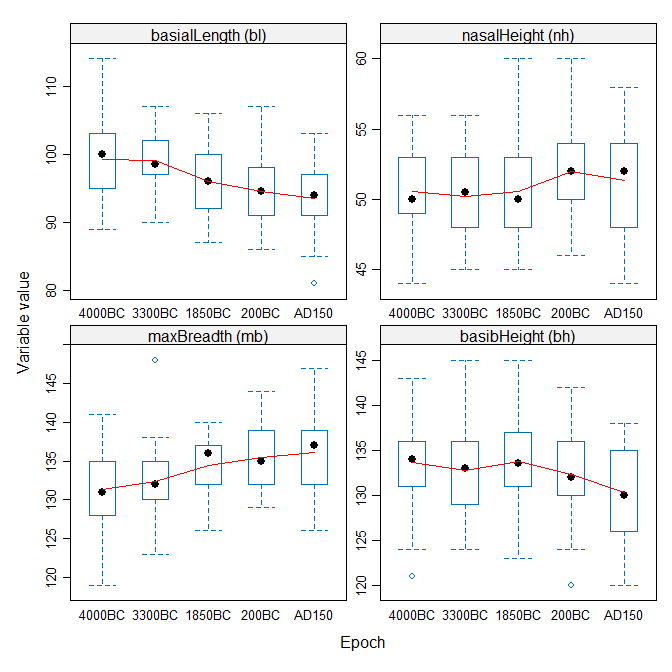Figure 3.3: Boxplots of Skulls data, by epoch, for each variable.

The trend lines aren’t linear, but neither are they random, so something systematic has been going on!

Now, fit the MANOVA model, and test the effect of epoch with car::Anova(). We see that the multivariate means differ substantially.

# fit manova model
sk.mod <- lm(cbind(mb, bh, bl, nh) ~ epoch, data=Skulls)
Anova(sk.mod)
#>
#> Type II MANOVA Tests: Pillai test statistic
#>       Df test stat approx F num Df den Df  Pr(>F)
#> epoch  4     0.353     3.51     16    580 4.7e-06 ***
#> ---
#> Signif. codes:  0 '***' 0.001 '**' 0.01 '*' 0.05 '.' 0.1 ' ' 1

Perhaps of greater interest are the more focused tests of trends over time. These are based on tests of the coefficients in the model sk.mod being jointly equal to zero, for subsets of the (polynomial) contrasts in epoch.

coef(sk.mod)
#>                    mb        bh        bl       nh
#> (Intercept) 133.97333 132.54667 96.460000 50.93333
#> epoch.L       4.02663  -2.19251 -5.017481  1.07517
#> epoch.Q      -0.46325  -1.26504 -0.089087  0.12472
#> epoch.C      -0.46380  -0.78003  1.075174 -0.83273
#> epoch^4       0.34263   0.80479 -0.661360 -0.41833

We use linearHypothesis() for a multivariate test of the epoch.L linear effect. The linear trend is highly significant. It is not obvious from Figure 3.2 that maximal breadth and nasal are increasing over time, while the other two measurements have negative slopes.

coef(sk.mod)["epoch.L",]
#>      mb      bh      bl      nh
#>  4.0266 -2.1925 -5.0175  1.0752
print(linearHypothesis(sk.mod, "epoch.L"), SSP=FALSE) # linear component
#>
#> Multivariate Tests:
#>                  Df test stat approx F num Df den Df    Pr(>F)
#> Pillai            1   0.29138   14.597      4    142 5.195e-10 ***
#> Wilks             1   0.70862   14.597      4    142 5.195e-10 ***
#> Hotelling-Lawley  1   0.41119   14.597      4    142 5.195e-10 ***
#> Roy               1   0.41119   14.597      4    142 5.195e-10 ***
#> ---
#> Signif. codes:  0 '***' 0.001 '**' 0.01 '*' 0.05 '.' 0.1 ' ' 1

linearHypothesis() can also be used to test composite hypotheses. Here we test all non-linear coefficients jointly. The result indicates that, collectively, all non-linear terms are not significantly different from zero.

print(linearHypothesis(sk.mod, c("epoch.Q", "epoch.C", "epoch^4")), SSP=FALSE)
#>
#> Multivariate Tests:
#>                  Df test stat approx F num Df den Df Pr(>F)
#> Pillai            3   0.06819  0.83726     12 432.00 0.6119
#> Wilks             3   0.93296  0.83263     12 375.99 0.6167
#> Hotelling-Lawley  3   0.07063  0.82791     12 422.00 0.6216
#> Roy               3   0.04519  1.62676      4 144.00 0.1707

Again, HE plots can show the patterns of these tests of multivariate hypotheses. With four response variables, it is easiest to look at all pairwise HE plots with the pairs.mlm() function. The statement below produces Figure 3.4. In this plot, we show the hypothesis ellipsoids for the overall effect of epoch, as well as those for the tests just shown for the linear trend component epoch.L as well as the joint test of all non-linear terms.

pairs(sk.mod, variables=c(1,4,2,3),
hypotheses=list(Lin="epoch.L",
NonLin=c("epoch.Q", "epoch.C", "epoch^4")),
var.labels=vlab[c(1,4,2,3)])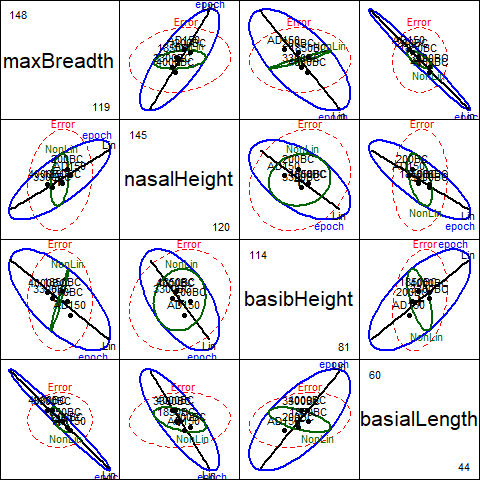Figure 3.4: Pairs HE plot of Skulls data, showing multivariate tests of epoch, as well as tests of linear and nonlinear trends.

These plots have an interesting geometric interpretation: the $$\mathbf{H}$$ ellipses for the overall effect of epoch are representations of the additive decomposition of this effect into
$$\mathbf{H}$$ ellipses for the linear and nonlinear linear hypothesis tests according to

$\mathbf{H}_{\textrm{epoch}} = \mathbf{H}_{\textrm{linear}} + \mathbf{H}_{\textrm{nonlinear}}$

where the linear term has rank 1 (and so plots as a line), while the nonlinear term has rank 3. In each panel, it can be seen that the large direction of the $$\mathbf{H}_{\textrm{epoch}}$$ leading to significance of this effect corresponds essentially to the linear contrast. $$\mathbf{H}_{\textrm{nonlinear}}$$ is the orthogonal complement of $$\mathbf{H}_{\textrm{linear}}$$ in the space of $$\mathbf{H}_{\textrm{epoch}}$$, but nowhere does it protrude beyond the boundary of the $$\mathbf{E}$$ ellipsoid.